# Selina Concise Solutions for Chapter 5 Playing with Numbers Class 8 ICSE Mathematics

##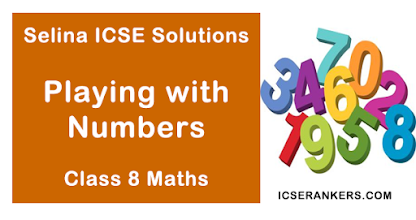### Exercise 5A

1. Write quotient when the sum of 73 and 37 is divided by
(i) 11
(ii) 10
Solution
Sum of 73 and 37 b is to be divided by
(i) 11
(ii) 10
Let ab = 73
and ba = 37
∴ a = 7
and b = 3
(i) The quotient of ab + bc i.e (73 + 37) when divided by 11 is a + b = 7 + 3 = 10
(ii) The quotient of ab + ba i.e. (73 + 37) when divided by 10 (i.e.  a + b ) is 11

2. Write the quotient when the sum of 94 and 49 is divide by
(i) 11
(ii) 13
Solution
Sum of 84 and 49 is to be divide by
(i) 11
(ii) 13
Let ab = 94
and ba = 49
∴ a = 9 and b = 4

(i) The quotient of 94 + 49 (i.e 9 + 4 = 13(ii) The quotient of 94 + 49 (i.e. ab + ba)
When divided by 13 i.e. (a + b) is 11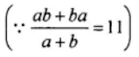3. Find the quotient when 73 – 37 is divide by
(i) 9
(ii) 4
Solution
Difference of 73 – 37 is to be divided by
(i) 9
(ii) 4
Let ab = 73 and ba = 37
∴ a = 7 and b = 3
(i) The quotient of 73 – 37 (i.e. ab – bc) when divided by 7 is  a - b i.e. 7 – 3 = 4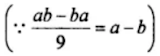(ii)
The quotient of 73 – 37 (i.e. ab – ba) when divided by 4 i.e. (a – b) is 94. Find the quotient when 94 – 49 is divided by
(i) 9
(ii) 5
Solution
Difference of 94 and 49 is divided by
(i) 9
(ii) 5
Let ab = 94 and ba = 49
∴ a = 9 and b = 4
(i) The quotient of 94 – 49 i.e. (ab – ba) when divided by 9 is (a – b) i.e. 9 – 4 = 5(ii) The quotient of 94 – 49 i.e. (ab – ba) when divide by 5 i.e.(a – b) is 95. Show that 527 + 752 + 275 is exactly divisible by 14.
Solution
Property:
abc = 100a + 106 + c ...(i)
bca = 1006 + 10c + a ...(ii)
and cab = 100c + 10a + b …(iii)
Adding, (i), (ii) and (iii), we get
abc + bca + cab = 111a + 111b + 111c = 111(a + b + c) = 3 × 37 (a + b + c)
Now, let us try this method on
527 + 752 + 275 to check is it exactly divisible by 14
Here, a = 5, 6 = 2, c = 7
527 + 752 + 275 = 3 × 37(5 + 2 + 7) = 3 × 37 × 14
Hence, it shows that 527 + 752 + 275 is exactly divisible by 14.

6. If a = 6, show that abc = bac.
Solution
Given: a = 6
To show: abc = bac
Proof:
abc = 100a + 106 + c ...(i)
(By using property 3)
bac = 1006 + 10a + c …(ii)
(By using property 3)
Since, a = 6
Substitute the value of a = 6 in equation (i) and (ii), we get
abc = 1006 + 106 + c …(iii)
bac = 1006  + 106 + c …(iv)
Subtracting (iv) from (iii) abc – bac = 0
abc = bac
Hence, proved.

7. if a > c; show that abc – cba = 99(a – c).
Solution
Given, a > c
To show : abc – cba = 99(a - c)
Proof:
abc = 100a + 10b + c …(i)
(By using property 3)
cba = 100c + 10b + a ...(i)
(By using property 3)
Subtracting, equation (ii) from (i), we get
abc – cba = 100a + c – 100c – a
⇒ abc – cba = 99a - 99c
⇒ abc – cba = 99(a – c)
Hence, proved.

8. If c > a; show that cba – abc = 99(c – a).
Solution
Given, c > a
To show : cba – abc = 99(c – a)
Proof:
cba = 100c + 106 + a …(i)
(by using property 3)
abc = 100a + 106 + c ...(ii)
(by using property 3)
Subtracting (ii) from (i)
cba – abc = 100c + 106 + a – 100a – 106c
⇒ cba – abc = 99c – 99a
⇒ cba – abc = 99(c – a)
Hence proved.

9. If a = c, show that cba- abc = 0
Solution
Given: a = c
To show: cba  - abc = 0
Proof:
cba = 100c + 106 + a …(i)
(By using property 3)
abc = 100a + 106 + c …(ii)
(By using property 3)
Since, a = c,
Substitute the value of a = c in equation (i) and (ii), we get
cba = 100c + 10b + c ...(iii)
abc = 100c + 10b  + c …(iv)
subtracting (iv) from (iii), we get
cba – abc – 100c + 106 + c – 100c – 106 – c
⇒ cba – abc = 0
⇒ cba = abc
Hence, proved.

10. Show that 954 – 459 is exactly divisible by 99.
Solution
To show: 954 – 459  is exactly divisible  by 399, where a = 9, b = 5, c = 4,
abc = 100a + 10b + c
⇒ 954 = 100 × 9 + 10 × 5 + 4
⇒ 954 = 900 + 50 + 4 ...(i)
And 459 = 100 × 4 + 10 × 5 + 9
⇒ 459  = 400 + 50 + 9 …(ii)
Subtracting (ii) from (i), we get
954 – 59 = 900 + 50 + 4 – 400 – 50 – 9
⇒ 954 – 459 = 500 – 5
⇒ 954 – 459 = 495
⇒ 954 – 458 = 99 × 5
Hence, 954 – 459 is exactly divisible by 99
Hence proved.

### Exercise 5 B

1.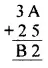Solution
A = 7 as 7 + 5 = 12.
We want 2 at units place and 1 is carry over. Now 3 + 2 + 1 = 6
B = 6
Hence, A = 7 and B = 6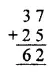2.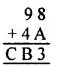Solution
A = 5 as 8 + 5 = 13.
We want 3 at units place and 1 is carry over. Now 9 + 4 + 1  = 14.
B = 4 and C = 1
Hence A = 5 and B = 4 and C = 13.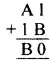Solution
B = 9 as 9 + 1 = 10.
We want 0 at units place and 1 is carry over. Now B – 1 – 1 = A.
∴  A = 9 – 2 = 7
Hence A = 7 and B = 9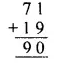4.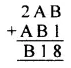Solution
B = 7 as 7 + 1 = 8. We want 8 at unit place.
Now,
7 + A = 11
∴  A = 11 – 7 = 4
Hence A = 4 and B = 75.Solution
A + B = 9
And 2 + A = 10
∴ A = 10 – 2= 8
And 8 + B = 9
∴ B = 9 – 8 = 1
Hence, A  = 8 and B = 1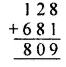6.Solution
As we need A at unit place and 9 at ten’s place,
A = 6 as 6 × 6 = 367.Solution
As we need B at unit place and B at ten’s place,
∴ B = 4 as 6 × 4 = 24
Now, we want to find A, 6 × A + 2 = 4 (at unit’s place)
∴ A = 78.Solution
As we need B at unit place and A at ten’s place,
∴ B = 0 as 3 × 0 = 0
Now, we want to find A, 3 × A (at unit’s place)
∴  A = 5, as 3 × 5 = 15
∴  C = 1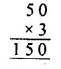9.Solution
As we need B at unit place and A at ten's place,
B = 0 as 5 × 0 = 0
Now, we want to find A, 5 ×  A = A (at unit's place)
A = 5, as 5 × 5 = 25
C = 2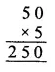10.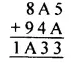Solution
5 + A = 13
And A + 4 = 13
∴ A = 13 – 5 = 8
Hence A = 811.Solution
C + 5 = 11
∴  C = 11 – 5 = 6
And 8 + B + 1 = 15
∴ B = 15 – 9 = 6
And A + 5 + 1 = 13
∴ A = 13 – 6 = 7
And 6 + D + 1 = 9
∴ D = 9 – 7 = 2
Hence A = 7,  B = 6, C = 6 and D = 2### Exercise 5 C

1. Find which of the following numbers are divisible by 2:
(i) 192
(ii) 1660
(iii) 1101
(iv) 2079
Solution
A number having its unit digit 2, 4, 6, 8 or 0 is divisible by 2.
So, Number 192, 1660 are divisible by 2.

2. Find which of the following numbers are divisible by 3:
(i) 261
(ii) 111
(iii) 6657
(iv) 2574
Solution
A number is divisible by 3 if the sum of its digits is divisible by 3,
So, 261, 111 are divisible by 3.

3. Find which of the following numbers are divisible by 4:
(i) 360
(ii) 3180
(iii) 5348
(iv) 7756
Solution
A number is divisible by 4, if the number formed by the last two digits is divisible by 4.
So, number 360, 5348, 7756 are divisible by 4.

4. Find which of the following numbers are divisible by 5 :
(i) 3250
(ii) 5557
(iii) 39255
(iv) 8204
Solution
A number having its unit digit 5 or 0, is divisible by 5.
So, 3250, 39255 are all divisible by 5.

5. Find which of the following numbers are divisible by 4:
(i) 360
(ii) 3180
(iii) 5348
(iv) 7756
Solution
A number having its unit digit 0, is divisible by 10.
So, 5100, 3400 are all divisible by 10.

6. Which of the following numbers are divisible by 11:
(i) 2563
(ii) 8307
(iii) 95635
Solution
A number is divisible by 11 if the difference of the sum of digits at the odd places and sum of the digits at even places is zero or divisible by 11.
So, 2563 is divisible by 11.

### Exercise 5 D

1. For what value of digit x, is :
1 × 5 divisible by 3?
Solution
1 × 5 is divisible by 3
⇒ 1 + x + 5 is  a multiple of 3
⇒ 6 + x = 0, 3, 6, 9
Since, x is a digit
x = 0, 3, 6 or 9

2. 31 × 5 is divisible by 3?
Solution
31 × 5 is divisible by 3
⇒ 3 + 1 + x + 5 is a multiple of 3
⇒ 9 + x = 0, 3, 6, 9,
⇒ x = -9 , -6, -3, 0, 3, 6, 9,
Since, x is a digit
x = 0, 3, 6 or 9

3. 28 × 6 is a multiple of 3?
Solution
28 × 6 is a multiple of 3
2 + 8 + x + 6 is  a multiple of 3
⇒ 16 + x = 0, 3, 6, 9, 12, 15,18
⇒ x  = -18, -5, -2, 0, 2, 5, 8
Since, x is a digit = 2, 5, 8

4. 24x divisible by 6?
Solution
24x is divisible by 6
⇒ 2 + 4 + x is a multiple of 6
⇒ 6 + x = 0, 6, 12
⇒ x = -6, 0, 6
Since, x is a digit
x = 0, 6

5. 3 × 26 a multiple of 6?
Solution
3 × 26 is a multiple of 6
3 + x + 2 + 6 is a multiple of 3
⇒ 11 + x = 0, 3, 6, 9, 12, 15, 18, 21
⇒ x = -11, -8, -5, -2, 1, 4, 7, 10, ……
Since, x is a digit
x = 1, 4 or 7

6. 42 × 8 divisible by 4?
Solution
42 × 8 is divisible by 4
⇒ 4 + 2 + x + 8 is a multiple of 2
⇒ 14 + x = 0, 2, 4, 6, 8,
⇒ x = -8, -6, -4, -2,  2, 4, 6, 8,
Since, x is a digit 2, 4, 6, 8

Question 7: 9142 × a multiple of 4?

Solution 7:
9142 × a multiple of 4
⇒ 9 + 1 + 4 + 2 + x is a multiple of 4
⇒ 16 + x = 0, 4, 8, ……..
x = -8, -4, 0, 4, 8
since, x is a digit 4, 8

8. 7 × 34 divisible by 9?
Solution
7 × 34 is multiple of 9
⇒ 7 + x + 3 + 4 is a multiple of 9
⇒ 14 + x = 0, 9, 18, 27,
⇒ x = -1, 4, 13,
Since, x is a digit x = 4

9. 5 × 555 a multiple of 9?
Solution
Sum of the digits of 5 × 555
= 5 + x + 5 + 5 + 5 = 20 + x
It is multiple by 9
The sum should be divisible by 9
Value of x will be 7

10. 3 × 2 divisible by 11?
Solution
Sum of the digit in even place = x
And sum of the  digits in odd place = 3 + 2 = 5
Difference of the sum of the digits in even places and in odd places = x – 5
3 × 2 is a multiple of 11
⇒ x – 5 = 0, 11, 22,
⇒ x = 5, 16, 27
Since, x is a digit x = 5

11. 5 × 2 is a multiple of 11?
Solution
Sum of digit in even place = x
And sum of the digits in odd place = 5 + 2 = 7
Difference of the sum of the digits in even places
And in odd places = x – 7
5 × 2 is a multiple of 11
⇒ x – 7 = 0, 11, 22
⇒ x – 7, 18, 29,
Since, x  is a digit x = 7